DISCLOSURE: This post may contain affiliate links, meaning when you click the links and make a purchase, we receive a commission.

# Excel Index Match single/multiple criteria with single/multiple results

In this article, we are going to discuss an important topic which is “Excel INDEX MATCH multiple criteria multiple results”. Finding the exact result for this is very difficult, so we are going to show different scenario for this. We are going to see that how the Index and Match functions can be used together to provide single/multiple results from multiple and single criteria. At the end of this article, you will get to see all possible combinations of INDEX and MATCH functions together.

## Using INDEX and MATCH Function with Multiple Criteria and Single Result

Let’s say we have a table containing various information about some people who are going to attend a conference. The table contains the information of the age, sex, country and ID of these people. Here we are going to use the INDEX and MATCH function together to collect the information of the ID of these people. We will see two processes of doing this. The table is given below.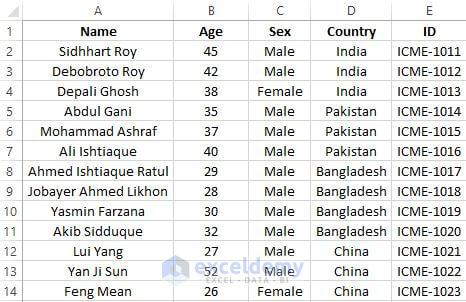### Process-1

In cell A16 of your worksheet, write down Name and in A17 write down ID. Now let’s say you want to find out the ID of Mr. Lui Yang from China. Write down his name in cell B16.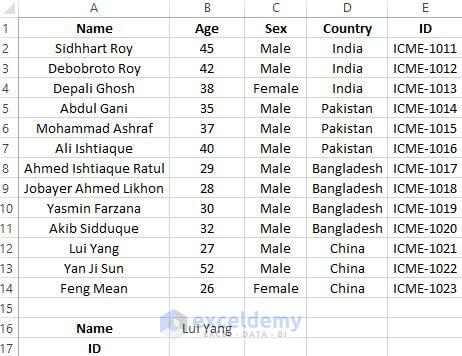In cell B17 write down the formula,`=INDEX(A2:E14,MATCH(B16,A2:A14,0),MATCH(A17,A1:E1,0))`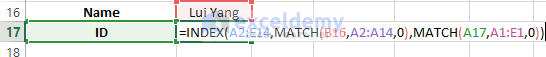The syntax of INDEX formula is, =INDEX(Reference, row_num, [column_num])

Here, with A2:E14 I defined the reference of the INDEX function. A2:A14 is the range where the name in cell B16 will be looked for and A1:E1 is the column headers where the cell A17 will be looked for. In this formula, the MATCH(B16,A2:A14,0) is the row_num argument and MATCH(A17,A1:E1,0) is the [column_num] argument of INDEX formula. After typing the formula in cell B17 you will get to see the below result.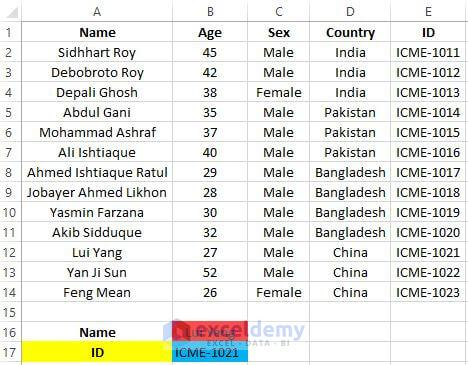From the above picture, you can see that we highlighted the cells B16, A17 and B17 with colors. Here the Red and Yellow filled cells which are B16 and A17 are the criteria of the INDEX and MATCH formula. It gives you the result in the blue filled cell which is cell B17. As I discussed above, the formula should find the ID number of Mr. Lui Yang. It performs the exact thing that I wanted to perform.

Note: By changing the text in cell A17 as Age, Sex and Country you will find the result of those based on the cell B16. Here the reference of INDEX function is the Name. If you want Country as a reference you must write Country in cell A17. Look into the below picture to get a nice idea about it.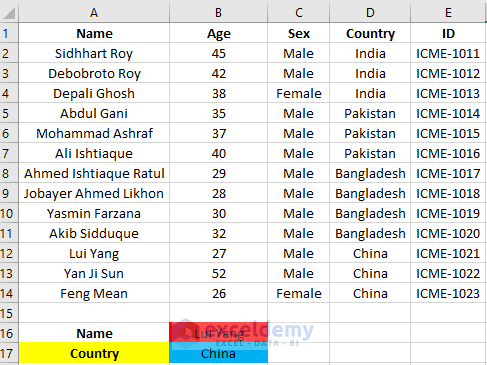### Process-2

From G4 to G7 write down Name, Sex, Country, and ID. In cell H4 to H6 write down these: Abdul Gani, Male, and Pakistan. In cell H6 write down the below formula, `=INDEX(E2:E14,MATCH(1,(H4=A2:A14)*(H5=C2:C14)*(H6=D2:D14),0))`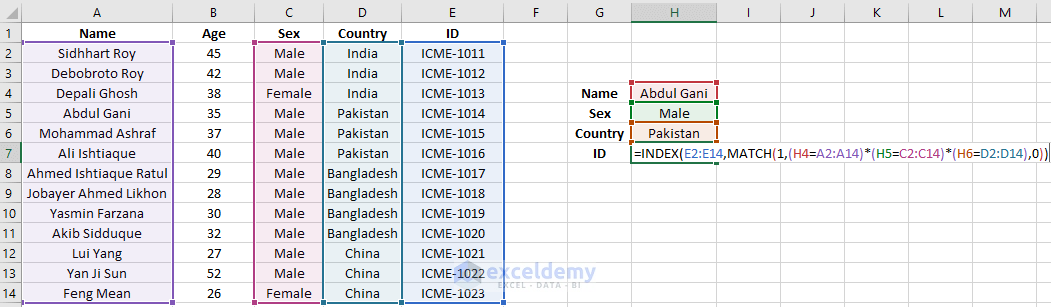After writing this formula press on to Ctrl + Shift + Enter together to get the result.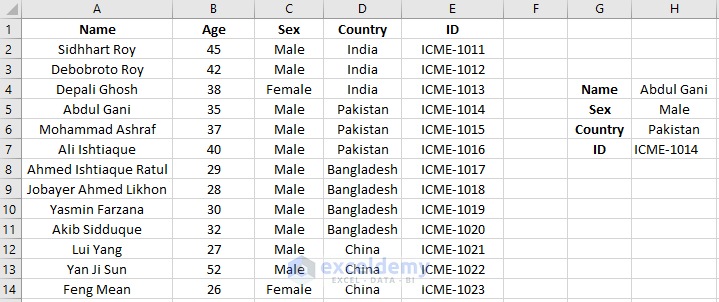## Excel Index Match for Single Criteria and Multiple Results

The INDEX and MATCH functions can be used to show multiple results with a single criterion. Here I will show you 2 processes whereby referencing Country`s name you are going to get the candidates name. Another one will show you how you can get all the information of a candidate based on his name only.

### Process-1

In cell G3, write down the country`s name from which you want to extract the names. Type this formula in cell H5, `=INDEX(\$A\$2:\$A\$14,SMALL(IF(ISNUMBER(MATCH(\$D\$2:\$D\$14,\$G\$3,0)),MATCH(ROW(\$D\$2:\$D\$14),ROW(\$D\$2:\$D\$14)),""),ROWS(\$F\$1:F1)))`

After typing this formula press Ctrl+Shift+Enter together. You will get to see the below result,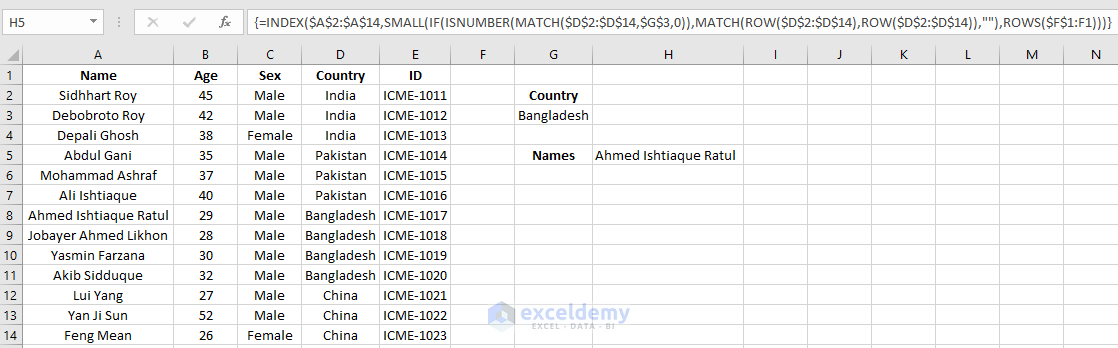Now drag down the formulated cell from H5 to H8 to get more results.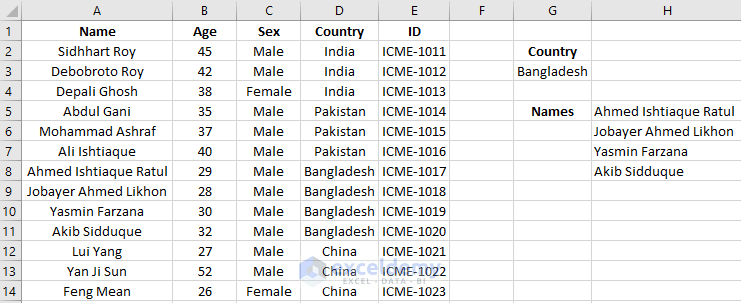Here you can see there are 4 Bangladeshi on the list. After inserting the formula with an array, you get the result of the first person only. Then by dragging the fill handle downwards gives you more names.

### Process-2

Here we will see how by referencing only names we get all information of the candidate like age, sex, country, and ID.  To perform this, write down Age, Sex, Country and ID in the range G5: G8. Write a candidate’s name in H3 and put a headline as Name in G3. Now in cell H5 write down the formula` =INDEX(\$A\$1:\$E\$14,MATCH(\$H\$3,(\$A\$1:\$A\$14),0),ROW()-3)`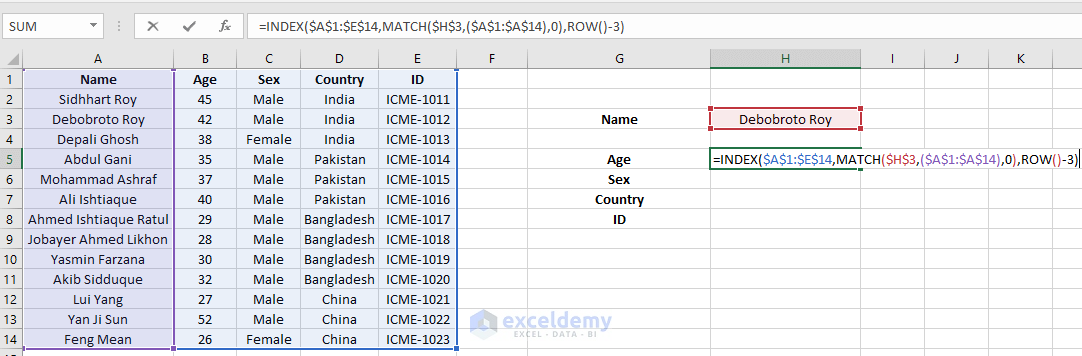After pressing Enter you will see the result 45. Now by dragging formulated cell downwards from H5 to H8, you will get to see the result.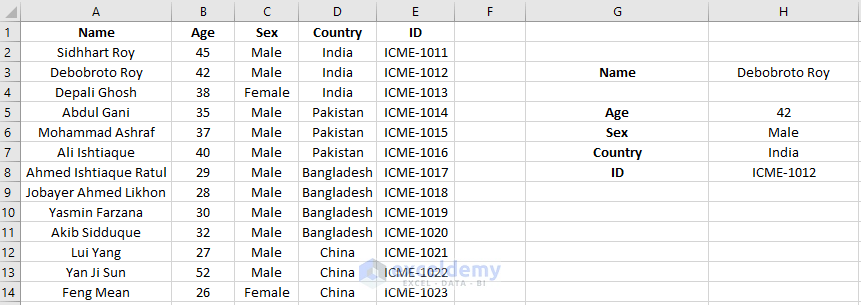To make it more exciting let`s do a data validation. Click on to cell H3 and pressing it to click on to the Data Validation option under the Data tab.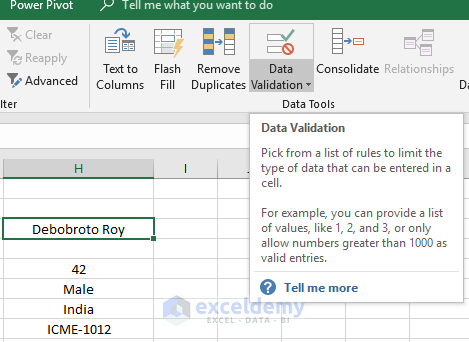In the Data Validation dialogue box select list and define the range just like the picture below.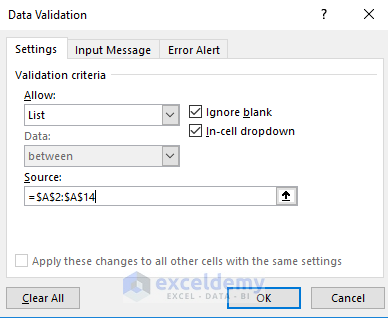After doing this press OK. You will see a drop-down option in cell H3. Now with this drop-down option, you can select any name to gather the required information using the formula.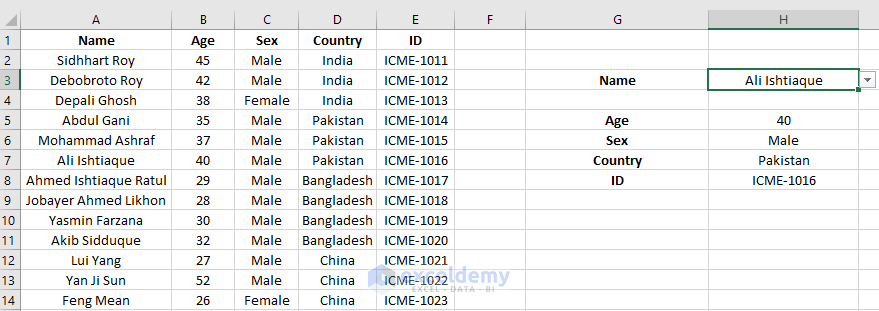## Using Excel Index Match with Multiple Criteria to Find Multiple Results

In this example, I will see how we can use multiple criteria as the reference in the INDEX and Match combined formula to find out multiple results. Let`s say you want to select 2 candidates based on their ID number. So, their ID numbers will be the criteria in this case for choosing them. In cell G4 and G5 write down two ID numbers and in cell H7, write down the formula, `=INDEX(\$A\$2:\$A\$14, SMALL(INDEX(MATCH(\$G\$4:\$G\$5, \$E\$2:\$E\$14, 0), ), ROWS(\$F\$1:F1)))`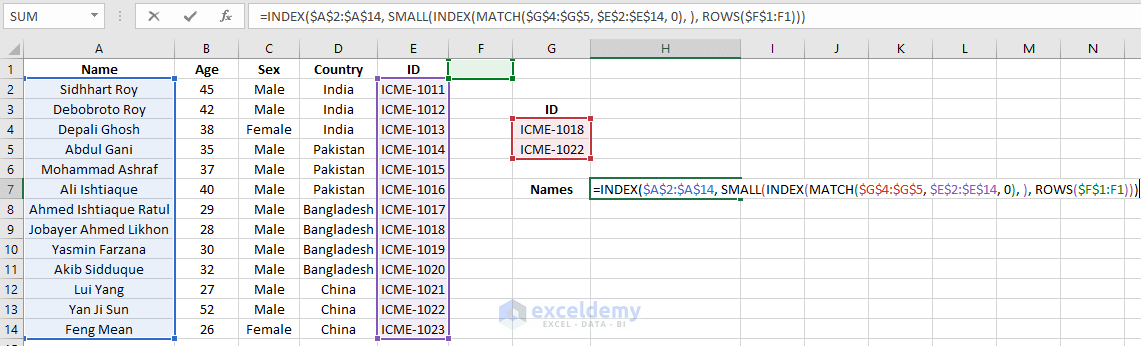Now after pressing Enter, you will get to see the below result.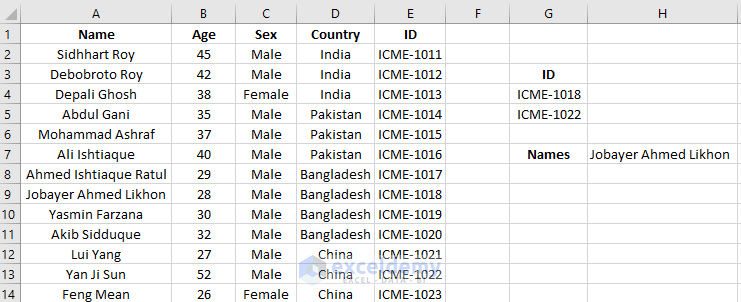Here the name which comes in cell H7 holds the ID ICME-1018. By dragging this formulated cell one cell downwards which is cell H8 here, you will get the candidates name who holds the ID no. ICME-1022. This is how you get multiple results with multiple criteria using the INDEX and MATCH function.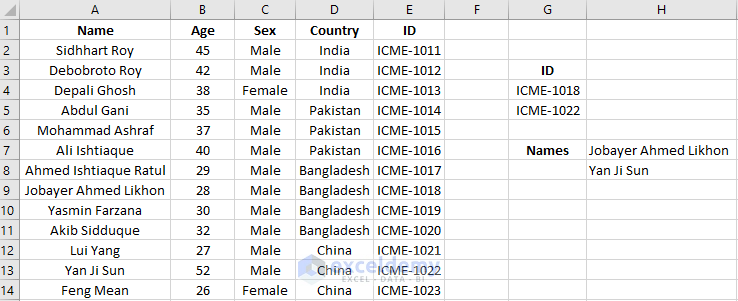Excel Index Match single/multiple criteria with single/multiple results

## Conclusion

The VLOOKUP function can be used instead of INDEX and MATCH functions. But the VLOOKUP functions have some limitations. INDEX and MATCH functions find different values based on some criteria in the whole worksheet. In case of VLOOKUP, it is not so easy to refer the whole cells at once. This article explains all possible combinations of INDEX and MATCH functions together. Hope you will like this article.

## Related Articles

#### Siam Hasan Khan

Hello! Welcome to my Profile. Here I will be posting articles related to Microsoft Excel. I have completed my BSc in Electrical and Electronic Engineering from American International University-Bangladesh. I am a diligent, goal-oriented engineer with an immense thirst for knowledge and attitude to grow continuously. Continuous improvement and life-long learning is my motto.

1 Comment